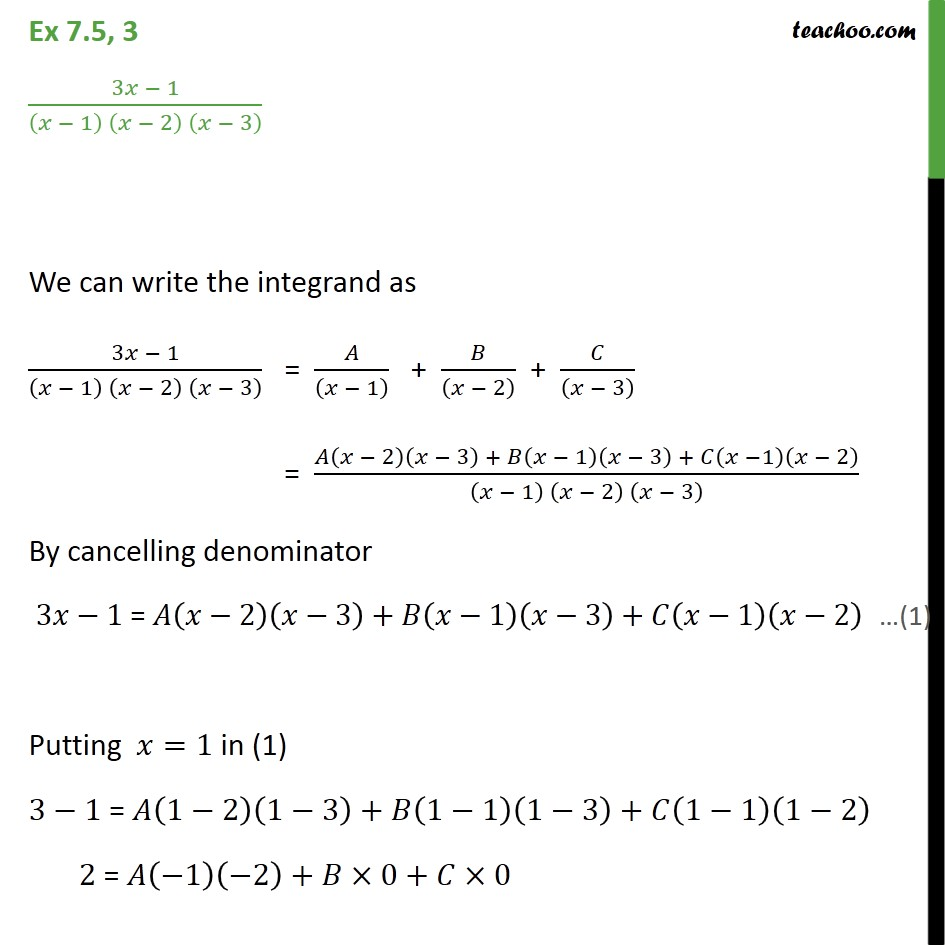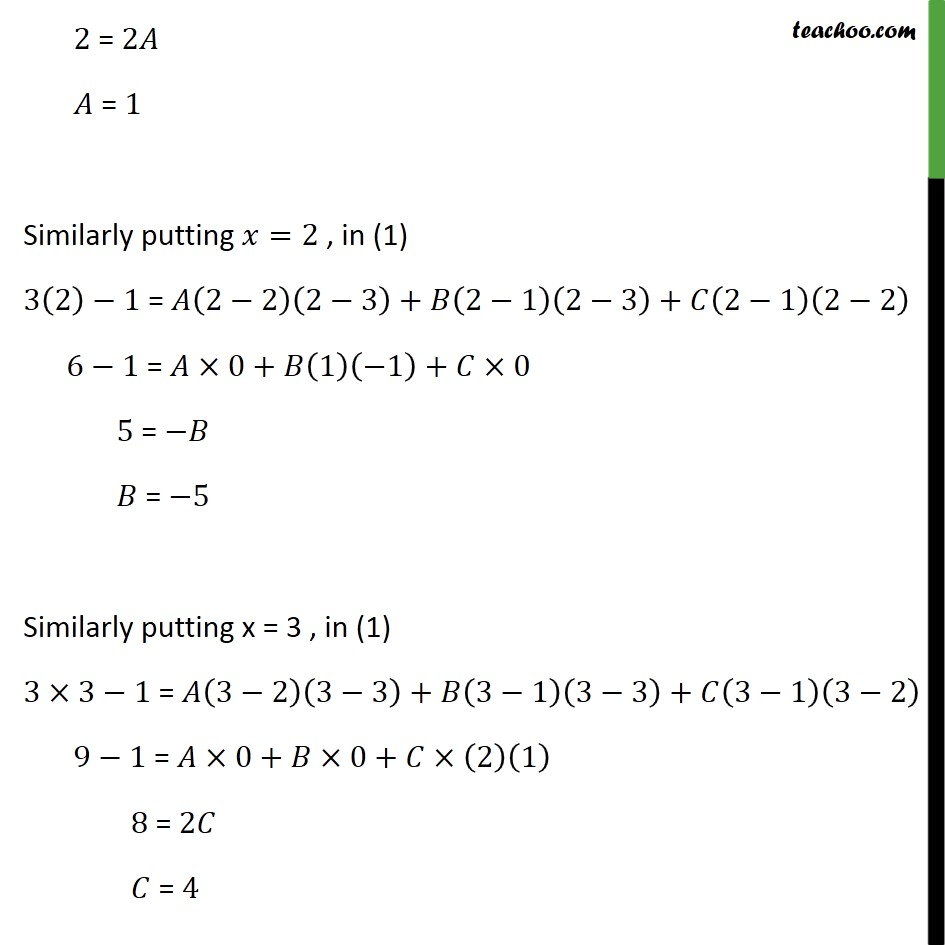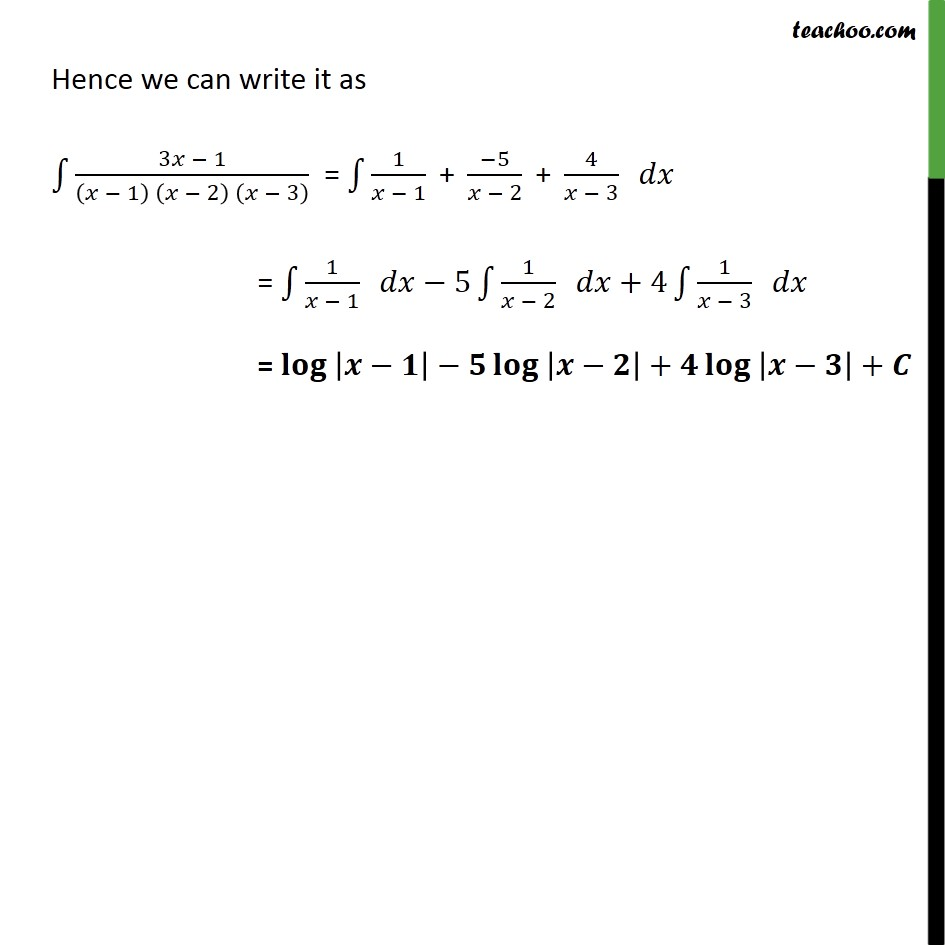Ex 7.5

Chapter 7 Class 12 Integrals
Serial order wiseLearn in your speed, with individual attention - Teachoo Maths 1-on-1 Class

### Transcript

Ex 7.5, 3 3 1 1 2 3 We can write the integrand as 3 1 1 2 3 = 1 + 2 + 3 = 2 3 + 1 3 + 1 2 1 2 3 By cancelling denominator 3 1 = 2 3 + 1 3 + 1 2 Putting =1 in (1) 3 1 = 1 2 1 3 + 1 1 1 3 + 1 1 1 2 2 = 1 2 + 0+ 0 2 = 2 = 1 Similarly putting =2 , in (1) 3 2 1 = 2 2 2 3 + 2 1 2 3 + 2 1 2 2 6 1 = 0+ 1 1 + 0 5 = = 5 Similarly putting x = 3 , in (1) 3 3 1 = 3 2 3 3 + 3 1 3 3 + 3 1 3 2 9 1 = 0+ 0+ 2 1 8 = 2 = 4 Hence we can write it as 3 1 1 2 3 = 1 1 + 5 2 + 4 3 = 1 1 5 1 2 +4 1 3 = + +